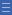# Linear models

Linear models

Linear models of fractions connect with students' concept of length. Equivalent fractions are recognised as being represented by equal lengths.

In linear models of fractions, the length of the whole is divided into equal lengths.

A fraction is identified as being a particular distance from the 'start' of the whole.

Dividing strips of paper into equal lengths is an example of using a linear model to represent fractions.

Two or more of these strips placed side-by-side allows comparison of different fractions and identification of equivalent fractions. For example, it could be observed that half a strip is the same length as two-quarters of a strip.

Placing fractions on a number line uses a similar approach, but highlights the fact that some fractions occupy the same position on a number line and therefore represent the same quantity.

Yes

Yes

Name Class SectionFraction strips Folder 17Fraction wall game Folder 17Year 4: Investigate equivalent fractions used in contexts Infobox 3Source Infobox 3